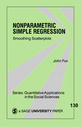Little Green Books

# Nonparametric Simple Regression

While regression analysis traces the dependence of the distribution of a response variable to see if it bears a particular (linear) relationship to one or more of the predictors, nonparametric regression analysis makes minimal assumptions about the form of relationship between the average response and the predictors. This makes nonparametric regression a more useful technique for analyzing data in which there are several predictors that may combine additively to influence the response. (An example could be something like birth order/gender/and temperament on achievement motivation). Unfortunately, researchers have not had accessible information on nonparametric regression analysis, until now. Beginning with presentation of nonparametric regression based on dividing the data into bins and averaging the response values in each bin, Fox introduces readers to the techniques of kernel estimation, additive nonparametric regression, and the ways nonparametric regression can be employed to select transformations of the data preceding a linear least-squares fit. The book concludes with ways nonparametric regression can be generalized to logit, probit, and Poisson regression.

locked icon

•••